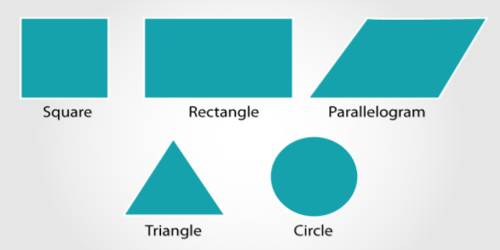Formula on Mensuration

Mensuration is the branch of mathematics which deals with the study of Geometric shapes, their area, volume and related parameters. Particularly it is the branch of geometry that deals with the measurement of length, area, or volume. It is based on the use of algebraic equations and geometric calculations to provide measurement data regarding the width, depth, and volume of a given object or group of objects.• Prism:

(i) Area of the lateral faces of a right prism = perimeter of the base × height.

(ii) Area of the whole surface of a right prism = Area of the lateral faces + 2 × area of the base.

(iii) A volume of a right prism = area of the base × height.

• Pyramid:

(i) Area of the lateral faces of a right pyramid = 1/2 × perimeter of the base x slant height.

(ii) Area of the whole surface of a right pyramid = area of the lateral faces + area of the base.

(iii) A volume of a right pyramid = 1/3 × area of the base × height.

• Tetrahedron:

If a be the length of an edge of a regular tetrahedron then

(i) the area of its slant sides = (3√3 / 4) a2 sq. units;

(ii) the area of its whole surface = √3a2 sq. units

(iii) the volume of the tetrahedron = √2/12 a3 cu. units.

Information Source: